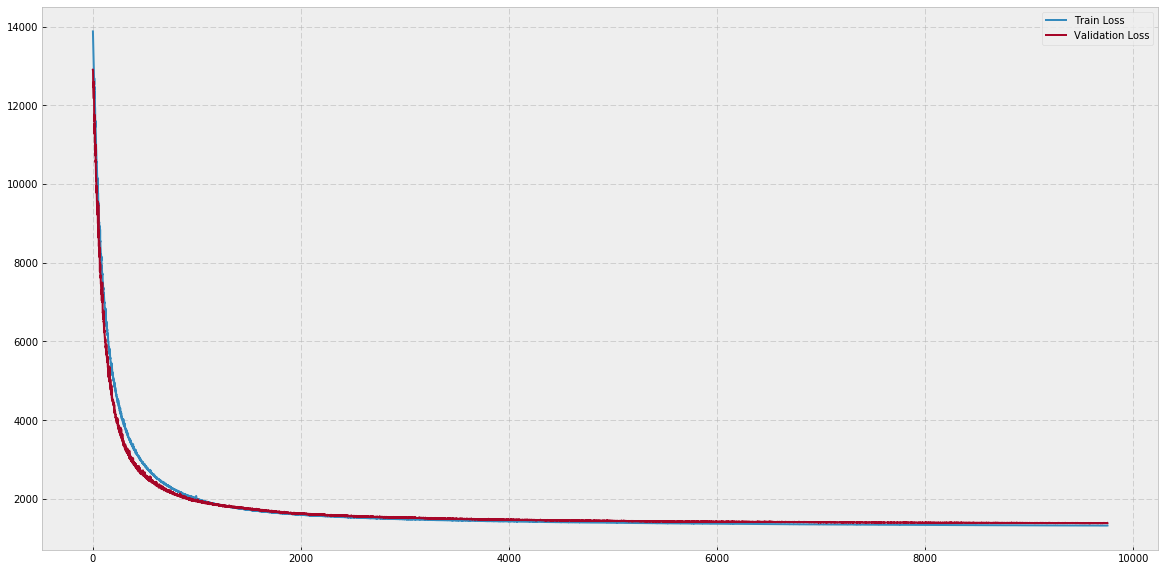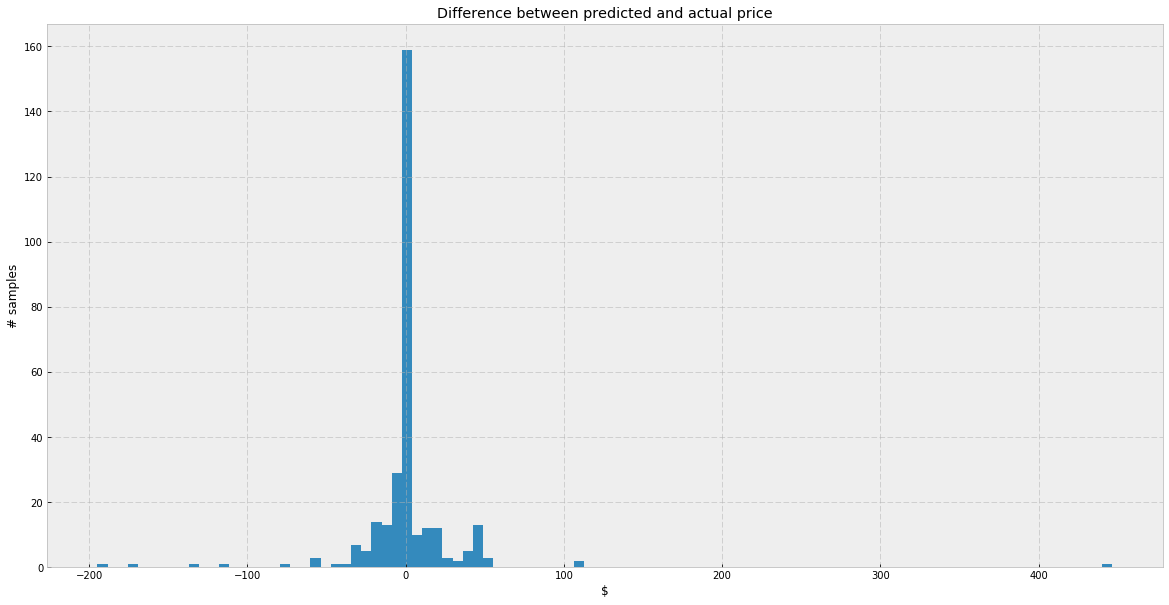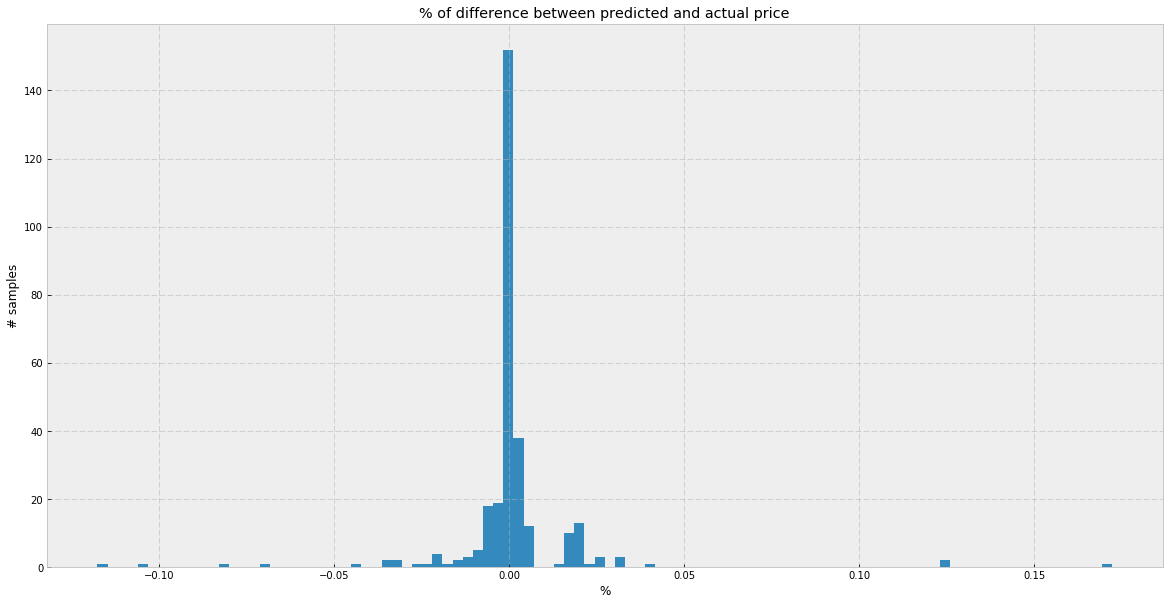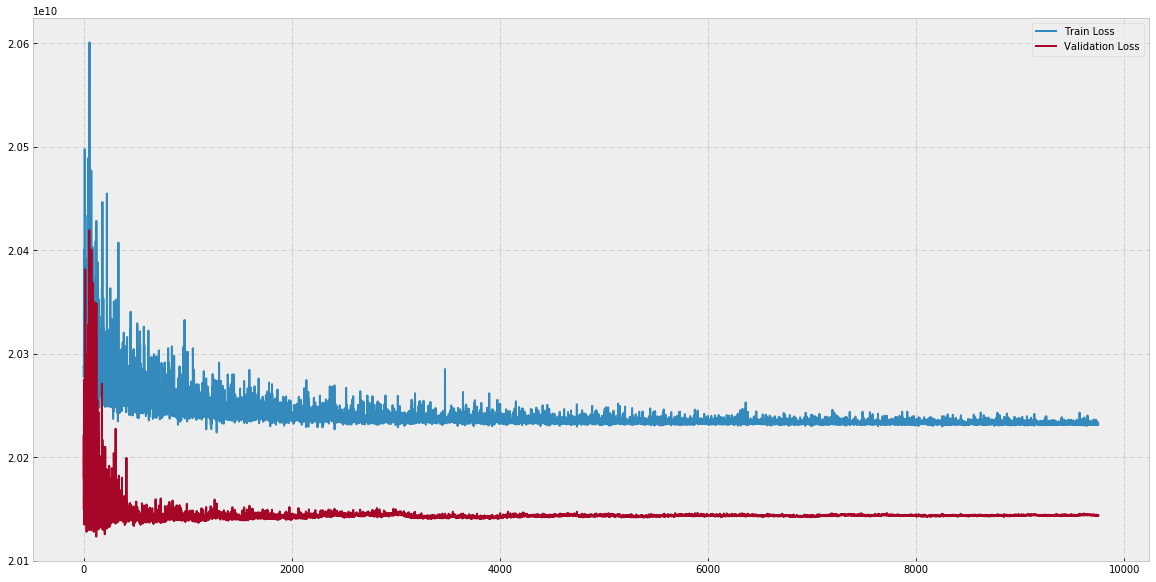# The purpose of this blog post:¶

1. To show how to implement (technically) a feature vector with both continuous and categorical features.
2. To use a Regression head to predict continuous values

We would like to predict the housing prices in 3 different suburbs of Tel Aviv (Mercaz, Old North and Florentine). Let's assume that a deterministic function $f(a, s, n)$ exist that determines the price of a house where:

• $n$: Number of rooms
• $s$: Size (in square meters)
• $a$: Area (TLV suburb)

For each area $a$ we define a price per square meter $s\_price(a)$ :

• Mercaz:     \$500 • Old North: \$350
• Florentine:     \$230 And an additional price per room$n\_price(a)$: • Mercaz: \$150,000
• Old North:     \$80,000 • Florentine: \$50,000

The price of a house in area $a$ with size $s$ and $n$ rooms will be: $$f(a, s, n) = s * s\_price(a) + n * n\_price(a)$$

This function is what we will later try to predict with a Regression DNN.

#### Imports and helper functions¶

In :
import numpy as np
import pandas as pd
import numpy as np
import pandas as pd
import keras
from keras.models import Sequential
from keras.layers import Dense, BatchNormalization
from keras.layers import Input, Embedding, Dense
from keras.models import Model
from keras.callbacks import Callback
import matplotlib.pyplot as plt

# Bayesian Methods for Hackers style sheet
plt.style.use('bmh')

np.random.seed(1234567890)

Using TensorFlow backend.

In :
class PeriodicLogger(Callback):
"""
A helper callback class that only prints the losses once in 'display' epochs
"""
def __init__(self, display=100):
self.display = display

def on_train_begin(self, logs={}):
self.epochs = 0

def on_epoch_end(self, batch, logs={}):
self.epochs += 1
if self.epochs % self.display == 0:
print ("Epoch: %d - loss: %f - val_loss: %f" % (self.epochs, logs['loss'], logs['val_loss']))

periodic_logger_250 = PeriodicLogger(250)


#### Define the mapping and a function that computes the house price for each example¶

In :
per_meter_mapping = {
'Mercaz': 500,
'Old North': 350,
'Florentine': 230
}

'Mercaz': 15. * 10**4,
'Old North': 8. * 10**4,
'Florentine': 5. * 10**4
}

def house_price_func(row):
"""
house_price_func is the function f(a,s,n).

:param row: dict (contains the keys: ['area', 'size', 'n_rooms'])
:return: float
"""
area, size, n_rooms = row['area'], row['size'], row['n_rooms']
return size * per_meter_mapping[area] + n_rooms * per_room_additional_price[area]


### Create toy data¶

In :
AREAS = ['Mercaz', 'Old North', 'Florentine']

def create_samples(n_samples):
"""
Helper method that creates dataset DataFrames

Note that the np.random.choice call only determines the number of rooms and the size of the house
(the price, which we calculate later, is deterministic)

:param n_samples: int (number of samples for each area (suburb))
:return: pd.DataFrame
"""
samples = []

for n_rooms in np.random.choice(range(1, 6), n_samples):
samples += [(area, int(np.random.normal(25, 5)), n_rooms) for area in AREAS]

return pd.DataFrame(samples, columns=['area', 'size', 'n_rooms'])


#### Create the train and validation sets¶

In :
train = create_samples(n_samples=1000)
val = create_samples(n_samples=100)


#### Calculate the prices for each set¶

In :
train['price'] = train.apply(house_price_func, axis=1)
val['price'] = val.apply(house_price_func, axis=1)


#### Here is the structure of the train/val DataFrame¶

In :
train.head()

Out:
area size n_rooms price
0 Mercaz 21 3 460500.0
1 Old North 26 3 249100.0
2 Florentine 27 3 156210.0
3 Mercaz 33 5 766500.0
4 Old North 23 5 408050.0

#### Define the features and the y vectores¶

We will separate the continuous and categorical variables

In :
continuous_cols = ['size', 'n_rooms']
categorical_cols = ['area']
y_col = ['price']

X_train_continuous = train[continuous_cols]
X_train_categorical = train[categorical_cols]
y_train = train[y_col]

X_val_continuous = val[continuous_cols]
X_val_categorical = val[categorical_cols]
y_val = val[y_col]


#### Normalization¶

In :
# Normalizing both train and test sets to have 0 mean and std. of 1 using the train set mean and std.
# This will give each feature an equal initial importance and speed up the training time
train_mean = X_train_continuous.mean(axis=0)
train_std = X_train_continuous.std(axis=0)

X_train_continuous = X_train_continuous - train_mean
X_train_continuous /= train_std

X_val_continuous = X_val_continuous - train_mean
X_val_continuous /= train_std


## Build a model using a categorical variable¶

### First let's define a helper class for the categorical variable¶

In :
class EmbeddingMapping():
"""
Helper class for handling categorical variables

An instance of this class should be defined for each categorical variable we want to use.
"""
def __init__(self, series):
# get a list of unique values
values = series.unique().tolist()

# Set a dictionary mapping from values to integer value
# In our example this will be {'Mercaz': 1, 'Old North': 2, 'Florentine': 3}
self.embedding_dict = {value: int_value+1 for int_value, value in enumerate(values)}

# The num_values will be used as the input_dim when defining the embedding layer.
# It will also be returned for unseen values
self.num_values = len(values) + 1

def get_mapping(self, value):
# If the value was seen in the training set, return its integer mapping
if value in self.embedding_dict:
return self.embedding_dict[value]

# Else, return the same integer for unseen values
else:
return self.num_values


### Create an embedding column for the train/validation sets¶

In :
area_mapping = EmbeddingMapping(X_train_categorical['area'])

X_train_categorical = X_train_categorical.assign(area_mapping=X_train_categorical['area'].apply(area_mapping.get_mapping))
X_val_categorical = X_val_categorical.assign(area_mapping=X_val_categorical['area'].apply(area_mapping.get_mapping))


#### A corresponding 'area_mapping' column was added to the train/validation sets¶

In :
X_train_categorical.head()

Out:
area area_mapping
0 Mercaz 1
1 Old North 2
2 Florentine 3
3 Mercaz 1
4 Old North 2

### Define the input layers¶

In :
# Define the embedding input
area_input = Input(shape=(1,), dtype='int32')

# Decide to what vector size we want to map our 'area' variable.
# I'll use 1 here because we only have three areas
embeddings_output = 1

# Let’s define the embedding layer and flatten it
area_embedings = Embedding(output_dim=embeddings_output, input_dim=area_mapping.num_values, input_length=1)(area_input)
area_embedings = keras.layers.Reshape((embeddings_output,))(area_embedings)

# Define the continuous variables input (just like before)
continuous_input = Input(shape=(X_train_continuous.shape, ))

# Concatenate continuous and embeddings inputs
all_input = keras.layers.concatenate([continuous_input, area_embedings])


### To merge them together we will use Keras Functional API¶

Will define a simple model with 2 hidden layers, with 25 neurons each.

In :
# Define the model
units=25
dense1 = Dense(units=units, activation='relu')(all_input)
dense2 = Dense(units, activation='relu')(dense1)
predictions = Dense(1)(dense2)

# Note using the input object 'area_input' not 'area_embeddings'
model = Model(inputs=[continuous_input, area_input], outputs=predictions)


#### Lets train the model¶

In :
epochs = 10000

# Note continuous and categorical columns are inserted in the same order as defined in all_inputs
history = model.fit([X_train_continuous, X_train_categorical['area_mapping']], y_train,
epochs=epochs, batch_size=128,
callbacks=[periodic_logger_250], verbose=0,
validation_data=([X_val_continuous, X_val_categorical['area_mapping']], y_val))

Epoch: 250 - loss: 14150.955505 - val_loss: 13591.749792
Epoch: 500 - loss: 4419.162722 - val_loss: 3791.042305
Epoch: 750 - loss: 2839.481758 - val_loss: 2596.868901
Epoch: 1000 - loss: 2283.344273 - val_loss: 2195.470615
Epoch: 1250 - loss: 1993.966924 - val_loss: 1953.627397
Epoch: 1500 - loss: 1827.210509 - val_loss: 1820.166925
Epoch: 1750 - loss: 1719.603118 - val_loss: 1749.322522
Epoch: 2000 - loss: 1644.947128 - val_loss: 1677.645994
Epoch: 2250 - loss: 1591.874908 - val_loss: 1623.897226
Epoch: 2500 - loss: 1555.967412 - val_loss: 1586.434529
Epoch: 2750 - loss: 1522.934362 - val_loss: 1563.956357
Epoch: 3000 - loss: 1498.848157 - val_loss: 1533.062053
Epoch: 3250 - loss: 1481.202623 - val_loss: 1530.011767
Epoch: 3500 - loss: 1461.785772 - val_loss: 1507.325862
Epoch: 3750 - loss: 1449.739195 - val_loss: 1490.356825
Epoch: 4000 - loss: 1435.963400 - val_loss: 1474.473166
Epoch: 4250 - loss: 1424.961645 - val_loss: 1469.804688
Epoch: 4500 - loss: 1418.407741 - val_loss: 1456.535400
Epoch: 4750 - loss: 1407.077398 - val_loss: 1450.191565
Epoch: 5000 - loss: 1397.807006 - val_loss: 1447.582760
Epoch: 5250 - loss: 1391.245334 - val_loss: 1440.799150
Epoch: 5500 - loss: 1384.866479 - val_loss: 1433.445653
Epoch: 5750 - loss: 1378.782277 - val_loss: 1428.455992
Epoch: 6000 - loss: 1373.368874 - val_loss: 1425.732157
Epoch: 6250 - loss: 1368.422633 - val_loss: 1416.285123
Epoch: 6500 - loss: 1361.978565 - val_loss: 1419.305588
Epoch: 6750 - loss: 1359.062253 - val_loss: 1416.092153
Epoch: 7000 - loss: 1354.401739 - val_loss: 1412.722853
Epoch: 7250 - loss: 1349.908637 - val_loss: 1405.402120
Epoch: 7500 - loss: 1345.809763 - val_loss: 1404.274998
Epoch: 7750 - loss: 1342.663633 - val_loss: 1400.794812
Epoch: 8000 - loss: 1340.327732 - val_loss: 1399.829547
Epoch: 8250 - loss: 1336.914749 - val_loss: 1391.678536
Epoch: 8500 - loss: 1334.828458 - val_loss: 1393.491002
Epoch: 8750 - loss: 1331.922844 - val_loss: 1391.898233
Epoch: 9000 - loss: 1328.771347 - val_loss: 1390.653604
Epoch: 9250 - loss: 1326.606613 - val_loss: 1385.063520
Epoch: 9500 - loss: 1324.080084 - val_loss: 1384.754880
Epoch: 9750 - loss: 1322.471232 - val_loss: 1381.195728
Epoch: 10000 - loss: 1321.835496 - val_loss: 1384.656379

In :
# Plot the train/validation loss values
plt.figure(figsize=(20,10))
_loss = history.history['loss'][250:]
_val_loss = history.history['val_loss'][250:]

train_loss_plot, = plt.plot(range(1, len(_loss)+1), _loss, label='Train Loss')
val_loss_plot, = plt.plot(range(1, len(_val_loss)+1), _val_loss, label='Validation Loss')

_ = plt.legend(handles=[train_loss_plot, val_loss_plot])After ~500 epochs the validation_loss is very low. This would have been a very good result if this was a "real world problem", particularly because we are using a quadratic loss function (Mean Square Error) when trying to predict a large value:

In :
print ("This is the average value we are trying to predict: %d" % y_val.mean().iloc)

This is the average value we are trying to predict: 288894


## How good are the model's predictions?¶

In :
df = y_val.copy()

# Add a column for the model's predicted values
df['pred'] = model.predict([X_val_continuous, X_val_categorical['area_mapping']])

# Calculate the difference between the predicted and the actual price
df['diff'] = df['pred'] - df['price']

# Calculate the absolute difference between the predicted and the actual price
df['abs_diff'] = np.abs(df['diff'])

# Calculate the percentage of the difference from the actual price
df['%diff'] = 100 * (df['diff'] / df['price'])

# Calculate the absolute percentage difference from the actual price
df['abs_%diff'] = np.abs(df['%diff'])


### What is the biggest difference in absolute values?¶

In :
# Sort by the 'abs_diff' field and show the 5 largest mistakes in absolute values

Out:
price pred diff abs_diff %diff abs_%diff
107 259200.0 259646.406250 446.406250 446.406250 0.172225 0.172225
13 165600.0 165405.203125 -194.796875 194.796875 -0.117631 0.117631
64 165950.0 165775.281250 -174.718750 174.718750 -0.105284 0.105284
163 166650.0 166515.453125 -134.546875 134.546875 -0.080736 0.080736
247 167000.0 166885.515625 -114.484375 114.484375 -0.068554 0.068554

The biggest absolute difference is ~\$537, which is less than a 0.25% of the ~\$240K house price

In :
# Calculate the mean and std. of the diff field
diff_mean, diff_std = df['diff'].mean(), df['diff'].std()
print("The mean is very close to 0 ({mean}) with std. {std}.".format(mean=round(diff_mean, 2), std=round(diff_std, 2)))

The mean is very close to 0 (1.53) with std. 37.24.

In :
# Here is the histogram of the differences
plt.figure(figsize=(20,10))
plt.hist(df['diff'], bins=100)
plt.xlabel("\$")
plt.ylabel("# samples")
_ = plt.title("Difference between predicted and actual price")### What is the biggest difference in percentage?¶

In :
# Sort by the '%diff' field and show the 5 largest proportional mistakes

Out:
price pred diff abs_diff %diff abs_%diff
107 259200.0 259646.406250 446.406250 446.406250 0.172225 0.172225
256 85600.0 85707.078125 107.078125 107.078125 0.125091 0.125091
94 85600.0 85707.078125 107.078125 107.078125 0.125091 0.125091
13 165600.0 165405.203125 -194.796875 194.796875 -0.117631 0.117631
64 165950.0 165775.281250 -174.718750 174.718750 -0.105284 0.105284

The biggest absolute/percentage difference comes from the same validation sample

In :
# Also, plot the histogram
plt.figure(figsize=(20,10))
plt.hist(df['%diff'], bins=100)
plt.xlabel("%")
plt.ylabel("# samples")
_ = plt.title("% of difference between predicted and actual price")## Let's try and define a model with only continuous variables¶

It is clear from the design of the problem that the housing prices cannot be correctly predicted without the categorical variable (area). Therefore, this model will surely fail to converge, and we expect the loss to stay high for both train/validation sets.

We will use the same simple architecture used with the embeddings model.

In :
continuous_input = Input(shape=(X_train_continuous.shape, ))

# Define the model
units=25
dense1 = Dense(units=units, activation='relu')(continuous_input)
dense2 = Dense(units, activation='relu')(dense1)
predictions = Dense(1)(dense2)

model_cont = Model(inputs=[continuous_input], outputs=predictions)

In :
#### Train the model
epochs = 10000

history_cont = model_cont.fit([X_train_continuous], y_train,
epochs=epochs, batch_size=128,
callbacks=[periodic_logger_250], verbose=0,
validation_data=([X_val_continuous], y_val))

Epoch: 250 - loss: 20299106041.855999 - val_loss: 20126572216.320000
Epoch: 500 - loss: 20256660488.192001 - val_loss: 20137029031.253334
Epoch: 750 - loss: 20278677695.146667 - val_loss: 20139098193.919998
Epoch: 1000 - loss: 20263610455.381332 - val_loss: 20139796616.533333
Epoch: 1250 - loss: 20258682869.077332 - val_loss: 20141625398.613335
Epoch: 1500 - loss: 20247740508.842667 - val_loss: 20142173143.040001
Epoch: 1750 - loss: 20238642124.117332 - val_loss: 20140987979.093334
Epoch: 2000 - loss: 20241048289.279999 - val_loss: 20142585255.253334
Epoch: 2250 - loss: 20238520718.677334 - val_loss: 20141372129.279999
Epoch: 2500 - loss: 20237267724.970665 - val_loss: 20143517750.613335
Epoch: 2750 - loss: 20234808781.482666 - val_loss: 20143563625.813332
Epoch: 3000 - loss: 20233952810.325333 - val_loss: 20145000611.840000
Epoch: 3250 - loss: 20243230545.237335 - val_loss: 20149649571.840000
Epoch: 3500 - loss: 20234373513.216000 - val_loss: 20141398070.613335
Epoch: 3750 - loss: 20236358860.799999 - val_loss: 20142996111.360001
Epoch: 4000 - loss: 20239934641.493332 - val_loss: 20141652459.520000
Epoch: 4250 - loss: 20232449130.495998 - val_loss: 20142593720.320000
Epoch: 4500 - loss: 20239852066.133335 - val_loss: 20143684048.213333
Epoch: 4750 - loss: 20234575066.453335 - val_loss: 20142832052.906666
Epoch: 5000 - loss: 20234614647.466667 - val_loss: 20143778365.439999
Epoch: 5250 - loss: 20233494069.248001 - val_loss: 20143480395.093334
Epoch: 5500 - loss: 20236085496.490665 - val_loss: 20143803351.040001
Epoch: 5750 - loss: 20233773023.231998 - val_loss: 20143587628.373333
Epoch: 6000 - loss: 20234148730.197334 - val_loss: 20144357894.826668
Epoch: 6250 - loss: 20231695548.416000 - val_loss: 20144359505.919998
Epoch: 6500 - loss: 20232812554.922668 - val_loss: 20144002990.080002
Epoch: 6750 - loss: 20233240231.936001 - val_loss: 20143567530.666668
Epoch: 7000 - loss: 20232301057.365334 - val_loss: 20143141355.520000
Epoch: 7250 - loss: 20233540504.234665 - val_loss: 20142721979.733334
Epoch: 7500 - loss: 20235362844.672001 - val_loss: 20143330317.653332
Epoch: 7750 - loss: 20233180976.469334 - val_loss: 20143820499.626667
Epoch: 8000 - loss: 20232677872.981335 - val_loss: 20143986578.773335
Epoch: 8250 - loss: 20232896195.242668 - val_loss: 20143647320.746666
Epoch: 8500 - loss: 20239680258.048000 - val_loss: 20144567528.106667
Epoch: 8750 - loss: 20237082542.080002 - val_loss: 20142775255.040001
Epoch: 9000 - loss: 20230405417.642666 - val_loss: 20143264727.040001
Epoch: 9250 - loss: 20231121141.759998 - val_loss: 20143636097.706665
Epoch: 9500 - loss: 20233319011.669334 - val_loss: 20143594100.053333
Epoch: 9750 - loss: 20235064410.112000 - val_loss: 20143228764.160000
Epoch: 10000 - loss: 20232211748.181332 - val_loss: 20143749611.520000

In :
# Plot the train/validation loss values
plt.figure(figsize=(20,10))
_loss = history_cont.history['loss'][250:]
_val_loss = history_cont.history['val_loss'][250:]

train_loss_plot, = plt.plot(range(1, len(_loss)+1), _loss, label='Train Loss')
val_loss_plot, = plt.plot(range(1, len(_val_loss)+1), _val_loss, label='Validation Loss')

_ = plt.legend(handles=[train_loss_plot, val_loss_plot])As expected the network failed to converge.

Note: To actually prove the network failed to converge, a much more rigorous test should be performed (grid of different architectures, learning rates and optimizers).